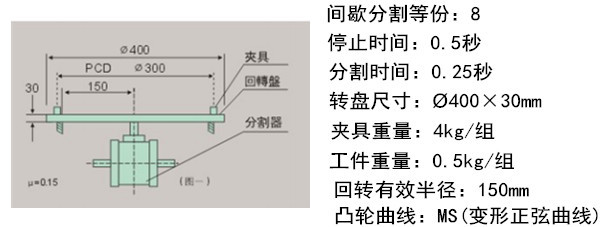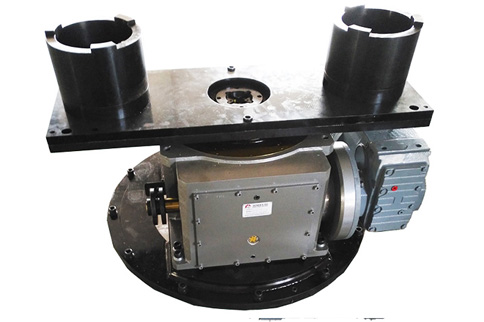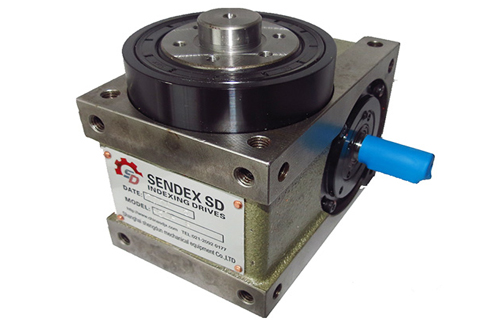# 凸轮分割器选型手册范例及计算公式1-1 间歇分割定位等份：S=8

1-2 转位角度θ=360°×0.25/（0.25+0.5）=360°×1/3=120°

1-3 入力轴之回转数：N=60/t1×θ/360=60/0.25×120/360=80rpm

1-4 凸轮曲线是变形正弦曲线，因此Vm=1.76，Am=5.53，Qm=0.987

1-5 负载安全系数fe=1.8

1-6 摩擦系数μ=0.2

(1)惯性扭力：Ti

（a）转盘重量：W1，夹具重量：W2,工件重量：W3，则： W1=л×20²×3×7.85×1/1000=29.59Kg

W2=4×8=32Kg W3=0.5×8=4Kg

（b）回转盘惯性矩：I1，夹具惯性矩：I2，工件惯性矩：I3，各为：

I1=W1R1²/2G=29.59×0.2²/2×9.8=0.060kgf.m.s²

I2=W2R2²/G=32×0.15²/9.8=0.0735kgf.m.s²

I3=W3R3²/G=4×0.15²/9.8=0.00992kgf.m.s²

（c）总惯性矩：I

I=I1+I2+I3=0.060+0.0735+0.0092=0.1427kgf.m.s²

因为是单道程，m=I

Ti=226.2Am×I.N²/S(θ/m)²=226.2×5.53×0.1427×80²/8×120²=9.916kgf.m

(2)摩擦扭矩：Tf

Tf=μ.W.R=0.2×(29.59+32+4)×0.15=1.968kgf.m

(3)工作扭矩：Tw

在间歇分割时没有作功，因此Tw=0

(4)以上总负载扭矩：Tt Tt=Ti+Tf+Tw=9.916+1.9968+0=11.884kgf.m

实际负载扭矩： Te=Tt.fe=11.884×1.8=21.391kgf.m

入力轴扭矩：Tc，注：入力轴起动负载扭矩视为0，因此Tca=0

Tc=360/θ.S×Qm×Te=360/(120×8)×0.987×21.391=7.917kgf.m

计算所需之马力，假设马达的效率为60%，则： P=Tc×N×9.8/9550×0.6=7.917×80×9.8/9550×0.6=1.083kw

事实上，以上所计算之值为起动时之最大马力，而连续传动所需之马力： Pa=P×1/2=1.083×1/2=0.542kw

(5)选择适用之间歇分割器

根据以上所计算之资料以及入力轴之转数80rpm来选择，请参考说明书上所记载，凡是出力轴扭矩高于以上所计算之Te值者均可选用。因为Te=21.391kgf.m，所以通过查询力矩表得知应该采用RU-110DF。

【相关推荐】### 关于平行凸轮分割器的机体的安装

2017-08-01### 弧面凸轮分割器的分类及选型

2017-08-01### 圣盾合作客户:江淮

2017-10-10

400 050 8006
• 服务热线

400 050 8006
• 微信扫一扫